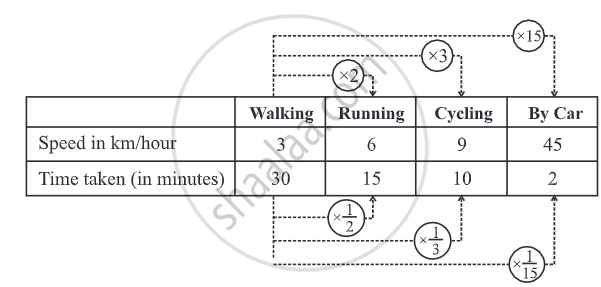# Concept of Inverse Proportion

#### notes

Inverse Proportion: Two quantities x and y are said to be in inverse proportion if Two quantities may change in such a manner that if one quantity increases, the other quantity decreases, and vice versa.

# Inverse Proportion:

• Two quantities x and y are said to be in inverse proportion if Two quantities may change in such a manner that if one quantity increases, the other quantity decreases, and vice versa.

• For example,
1) As the number of workers increases, the time taken to finish the job decreases.
2) If we increase the speed, the time taken to cover a given distance decreases.

• To understand this, let us look into the following situation.
Zaheeda can go to her school in four different ways. She can walk, run, cycle, or go by car. As Zaheeda doubles her speed by running, time reduces to half. As she increases her speed to three times by cycling, time decreases to one third. Similarly, as she increases her speed to 15 times, time decreases to one-fifteenth. (Or, in other words, the ratio by which time decreases is inverse of the ratio by which the corresponding speed increases).## Relation for Inverse Proportion:

Two quantities x and y are said to be in inverse proportion if an increase in x causes a proportional decrease in y (and vice-versa) in such a manner that the product of their corresponding values remains constant. That is, if xy = k, then x and y are said to vary inversely. In this case if y1, y2 are the values of y corresponding to the values x1, x2 of x respectively then x1y1 = x2y2 or x_1/x_2 = y_2/y_1.

#### Example

6 pipes are required to fill a tank in 1 hour 20 minutes. How long will it take if only 5 pipes of the same type are used?

Let the desired time to fill the tank be x minutes. Thus, we have the following table.
 Number of pipes 6 5 Time (in minutes) 80 x
Lesser the number of pipes more will be the time required by it to fill the tank. So, this is a case of inverse proportion.
Hence, 80 × 6 = x × 5.......[x1y1 = x2y2]
(80 xx 6)/5 = x
x = 96
Thus, time taken to fill the tank by 5 pipes is 96 minutes or 1 hour 36 minutes.

#### Example

If 15 workers can build a wall in 48 hours, how many workers will be required to do the same work in 30 hours?
Let the number of workers employed to build the wall in 30 hours be y.
 Number of hours 48 30 Number of workers 15 y
Obviously more the number of workers, the faster will they build the wall.
So, the number of hours and number of workers vary in inverse proportion.
So 48 × 15 = 30 × y
Therefore, (48 xx 15)/30 = y
y = 24
i.e., to finish the work in 30 hours, 24 workers are required.
If you would like to contribute notes or other learning material, please submit them using the button below.

### Shaalaa.com

Concept of Inverse Proportion [00:08:38]
S
0%